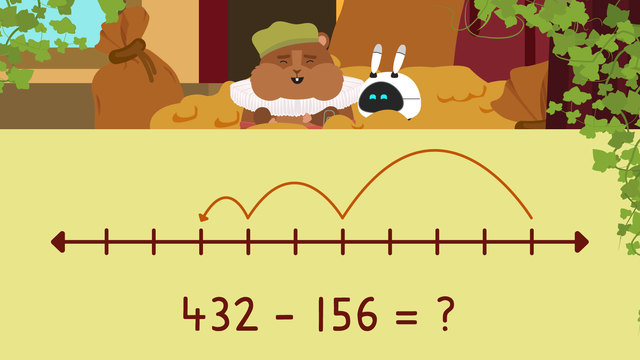# Three Digit Subtraction with Regrouping - Number LineRate this video

Ø 5.0 / 1 ratings

The authorTeam Digital
Three Digit Subtraction with Regrouping - Number Line
CCSS.MATH.CONTENT.3.NBT.A.2

## Information about the videoThree Digit Subtraction with Regrouping - Number Line

### In This 3rd Grade Video On 3 Digit Subtraction Using A Number Line

Sir Squeaks and Imani have jumped back in their time machine and headed to the Middle Ages where they meet King Theodore. He is extremely upset because someone has stolen from his vault! Hundreds of jewels and coins are missing. However, he doesn’t even know how many were taken. In this video, Sir Squeaks and Imani learn to use number line strategy with subtraction of 3-digit numbers to help calculate differences.

### 3 Digit Subtraction On An Open Number Line

To help solve subtraction equations with regrouping, we can model with an open number line. Open number lines are helpful because they allow us to see the movement while taking away an amount during subtraction.We will use four hundred thirty two minus one hundred fifty six to illustrate how to use open number line subtraction with 3 digits. First, draw a line and plot the larger number in the equation on the right side.Then, write the second numbers in the equation, or amount we are taking away, in expanded form. One hundred fifty-six becomes one hundred plus fifty plus six.Beginning with the hundreds, count back each hundred and plot the answer, three hundred thirty two.Then, move to the tens and skip count back for each ten. Plot the answer, two hundred eighty two.Finally, move to the ones and count back for each of the ones. Two hundred seventy-six is the number we end up on, so this is our solution!### 3 Digit Subtraction with a Number Line: Summary of Steps

• First, draw the number line and plot the larger number in the equation on the right side.

• Next, write the second number in the equation, or the amount taken away, in expanded form.

• Next, skip count back by the hundreds, tens, then ones of the second number, plotting the answers as you go.

• The final number you land on is the solution.

### 3 Digit Subtraction Open Number Line Worksheets With Friendly Numbers

Have you practiced 3 digit subtraction on number line worksheets yet? On this website, you can also find 3 digit subtraction open number line worksheets and exercises.

### TranscriptThree Digit Subtraction with Regrouping - Number Line

Sir Squeaks and Imani have traveled back in time to the Middle Ages where they meet King Theodore. He is extremely upset because someone has stolen from his vault! Many jewels and coins are missing. He doesn’t know how many were taken, so Sir Squeaks and Imani will help him using "Three Digit Subtraction with Regrouping- Number Line". Remember, number lines are useful tools that help us model equations. We can use an open number line to see the movement while taking away an amount during subtraction. Let's use four hundred thirty two minus one hundred fifty six as an example. First, draw a line and plot the larger number on the right side. Then, write the amount we are taking away in expanded form. One hundred fifty-six becomes one hundred plus fifty plus six. Beginning with the hundreds, count back each hundred and plot the answer, three hundred thirty two. Then, move to the tens, skip count back for each ten, and plot the answer, two hundred eighty two. Finally, move to the ones and count back for each of the ones. Two hundred seventy-six is the number we end up on so this is our solution! King Theodore knows that he had two hundred fifteen jewels now two hundred and six remain. Let's use a number line to help calculate how many were taken. Begin by drawing our number line and plotting two hundred fifteen on the right side. Next, we write two hundred and six in expanded form: two hundred plus zero plus six. Beginning with the hundreds, count back one hundred, then two hundred and plot the answer, fifteen. Move to the tens since there are no tens we have nothing to count so we move on to the ones and count back one, two, three, four, five, six! Plot nine since we finished there. Nine is our solution. Sir Squeaks reports that nine jewels were stolen from the vault. King Theodore also had five hundred twenty-five coins and now four hundred eighty-five are left. What are our steps? First draw the number line and plot five hundred twenty-five on the right side. Second, write four hundred eighty five in expanded form: four hundred plus eighty plus five. Now begin to count back for each hundred one hundred, two hundred, three hundred, four hundred and write the answer, one hundred twenty five. Where do we count next? We count the tens and count back eight tens until we reach and plot forty five! What happens next? We count back by the ones one, two, three, four, five! We finished on forty so write it here. Forty is our solution. So, Imani reports that forty coins were taken from the vault! Wait, who is this? Before we find out, let's summarize! Remember, to help solve subtraction equations with regrouping, we can model with an open number line. First, draw the number line and plot the larger number on the right side. Second, write the amount taken away in expanded form. Third, skip count back by the hundreds, tens, then ones, plotting the answers as you go. The final number is the solution. "I'm so glad you're back. There has been a theft!" "About that....you see, I was trying out a new invisibility spell this morning, when I was called away for an emergency. But I'm back now and it's as simple as..."